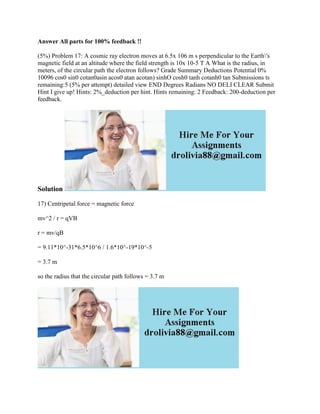Successfully reported this slideshow.

# Answer All parts for 100- feedback !! (5-) Problem 17- A cosmic ray el.docx×

# Answer All parts for 100- feedback !! (5-) Problem 17- A cosmic ray el.docx

Answer All parts for 100% feedback !!
(5%) Problem 17: A cosmic ray electron moves at 6.5x 106 m s perpendicular to the Earth\'s magnetic field at an altitude where the field strength is 10x 10-5 T A What is the radius, in meters, of the circular path the electron follows? Grade Summary Deductions Potential 0% 10096 cos0 sin0 cotan0asin acos0 atan acotan) sinhO cosh0 tanh cotanh0 tan Submissions ts remaining:5 (5% per attempt) detailed view END Degrees Radians NO DELI CLEAR Submit Hint I give up! Hints: 2%_deduction per hint. Hints remaining: 2 Feedback: 200-deduction per feedback.
Solution
17) Centripetal force = magnetic force
mv^2 / r = qVB
r = mv/qB
= 9.11*10^-31*6.5*10^6 / 1.6*10^-19*10^-5
= 3.7 m
so the radius that the circular path follows = 3.7 m
.

Answer All parts for 100% feedback !!
(5%) Problem 17: A cosmic ray electron moves at 6.5x 106 m s perpendicular to the Earth\'s magnetic field at an altitude where the field strength is 10x 10-5 T A What is the radius, in meters, of the circular path the electron follows? Grade Summary Deductions Potential 0% 10096 cos0 sin0 cotan0asin acos0 atan acotan) sinhO cosh0 tanh cotanh0 tan Submissions ts remaining:5 (5% per attempt) detailed view END Degrees Radians NO DELI CLEAR Submit Hint I give up! Hints: 2%_deduction per hint. Hints remaining: 2 Feedback: 200-deduction per feedback.
Solution
17) Centripetal force = magnetic force
mv^2 / r = qVB
r = mv/qB
= 9.11*10^-31*6.5*10^6 / 1.6*10^-19*10^-5
= 3.7 m
so the radius that the circular path follows = 3.7 m
.